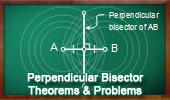Perpendicular Bisector, Theorems and Problems: Table of ContentPerpendicular lines.
Teorems and Problems.
Perpendicular Bisector.

Midpoints.
Theorems and Problems

Isosceles Triangle.
Perpendicular Bisector.

Rhombus.
The diagonals of a rhombus are perpendicular bisectors of one another.

Circumcenter. Index.
The circumcenter of a triangle is the intersection of any two of the three perpendicular bisectors.

GeoGebra, Dynamic Geometry: Circumcenter and Circumcircle of a Triangle.
HTML5 Animation for Tablets (iPad, Nexus..)

Congruence. Index.
Any point on a perpendicular bisector of a segment is equidistant from the endpoints of the segment.

Carnot's Theorem in an Acute Triangle.
Perpendicular Bisectors.

Carnot's Theorem in an Obtuse Triangle.
Perpendicular Bisectors.

Geometry Problem 1441.
Intersecting Circles, Perpendicular Bisector, Parallel Lines, Art, Poster, Typography, iPad Apps.

Geometry Problem 1440.
Intersecting Circles, Perpendicular Bisector, Collinear Points, Art, Poster, Typography, iPad Apps.

Geometry Problem 1419.
Triangle, Altitude, Circumcircle, Incenter, Inradius, Perpendicular, Angle Bisector, Midpoint.

Geometry Problem 1400.
Triangle with three rectangles on the sides, Perpendicular Bisectors, Concurrency.

Geometry Problem 1348.
Three Concentric Circles, Equilateral Triangle, Perpendicular Bisector, Congruence.

Geometry Problem 1201
Triangle, Interior Perpendicular Bisector, Midpoint, Area.

Geometry Problem 1032: Apollonius Problem LPP.
Circle Tangent to One Line and passing through Two Points.

Geometry Problem 1142.
Cyclic Quadrilateral, Isogonal Lines, Circle, Center, Radius, Perpendicular Bisector, Circumcenter.

Geometry Problem 1113.
Triangle, Perpendicular Bisector, 90 Degrees, Circle, Concyclic Points, Cyclic Quadrilateral.

Geometry Problem 1004.
Triangle with Squares, Perpendicular Bisectors, Concurrent Lines.

Geometry Problem 1001.
Triangle, Circumcircle, Perpendicular, Perpendicular Bisector, Tangent, Collinear Points.

Geometry Problem 995.
Quadrilateral, Perpendicular Diagonals, Perpendicular Bisector, Parallel.

Geometry Problem 859
Isosceles Right Triangle, Angles,18, 45 Degrees, Congruence, Perpendicular bisector.

Geometry Problem 663.
Scalene Triangle, 60 Degrees, Altitude, Angle Bisector, Perpendicular Bisector.

Geometry Problem 842
Triangle, Medians, Centroid, Four Circumcenters, Perpendicular, Congruence, Similarity.

Geometry Problem 841
Triangle, Medians, Centroid, Circumcenters, Perpendicular, Congruence, Similarity.

Problem 538.
Triangle, Perpendicular Bisector, Circumcircle, Midpoint.

Proposed Problem 401.
Area, Triangle, Angle bisector, Circumcircle, Perpendicular bisector, Congruence.

Proposed Problem 400.
Triangle, Angle bisector, Circumcircle, Perpendicular, Congruence.

Proposed Problem 78: Angles of a Circle. Perpendicular and parallel lines, Midpoint, Diameter, Chord, Cyclic quadrilateral, Congruence, Perpendicular Bisector.

Home | SearchGeometry | Lines | Post a comment | Email | By Antonio Gutierrez
Last updated Jul 5, 2019